# How to Convert yyyymmdd to Normal Date Format in Excel?

Sometimes in Excel, you can see that the dates in the sheet have no separators between the date, month, and year. So, we need to convert them to a valid fSometimes in Excel, you can see that the dates in the sheet have no separators between the date, month, and year. So, we need to convert them to a valid format to perform any operations on them and to make our sheet more efficient. If we try to solve this problem using the format cells, then an error will be displayed in the result. So, we need to use other methods to convert them into a valid format.

Read this tutorial to learn how you can convert YYYY-MM-DD to a normal name in Excel.

## Converting "yyyymmdd" to Normal Date Format with Formula

Here, we will first use the formula to get any one of the results, then use the auto-fill handle to get all the results. Let's look at a simple procedure for converting "yyyymmdd" to a normal date format in Excel.

### Step 1

Consider an Excel sheet with a list of dates in "yyyymmdd" format, similar to the image below.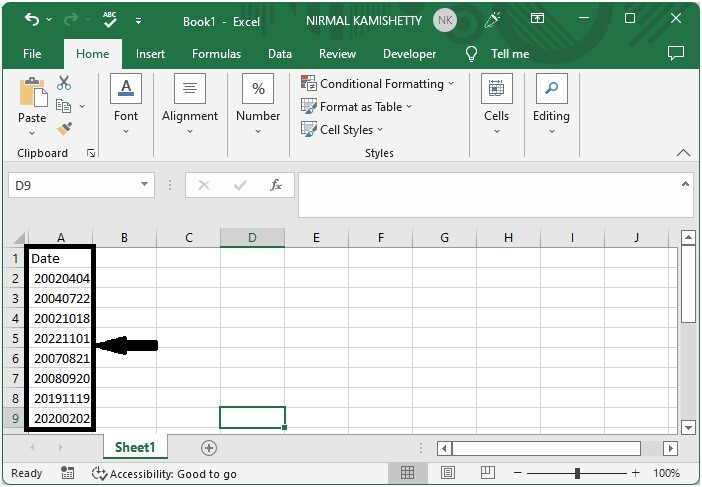To get our first result, click on an empty cell, in this case cell B2, and enter the formula =DATE(LEFT(A2,4),MID(A2,5,2),RIGHT(A2,2) and press enter. In the formula, A2 is the address of our value.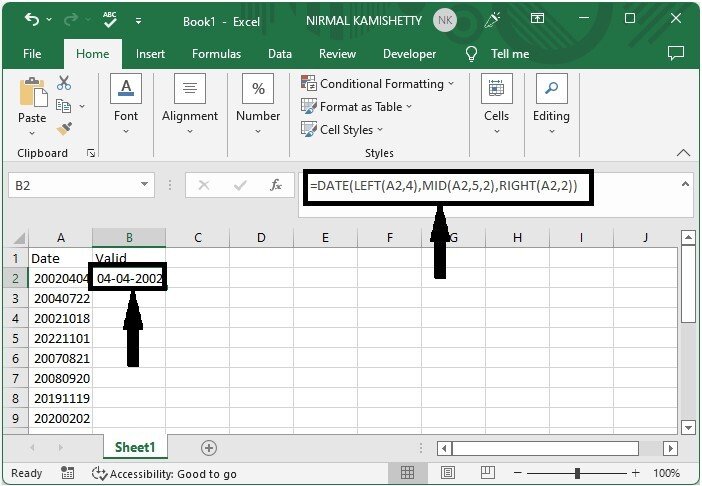### Step 2

Now to get all the results, drag down from the first result using the auto-fill handle, and our final result will be similar to the below image.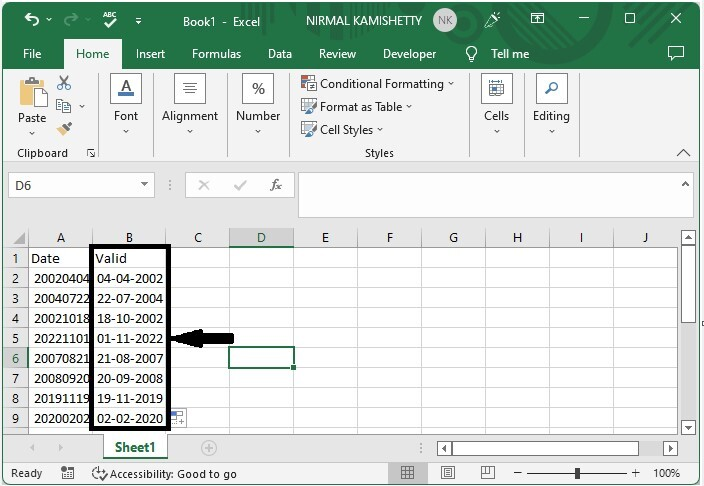## Converting "yyyymmdd" to Normal Date Format

Here we will use the Text to Column function in Excel. Let's go over a simple procedure for converting yyyymmdd to normal date format in Excel using the text-to-column function.

### Step 1

Let us consider the same data which used in above example.

Then select the values and click on data, then select text to column and click on delimited, then click on next.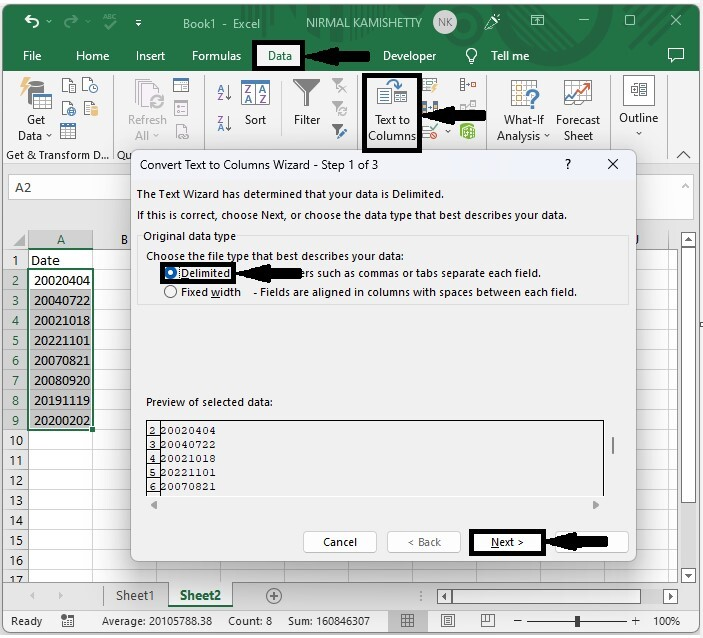### Step 2

Then click on "Next" and go to step 3, then click on "Date" and "Finish" to complete our process.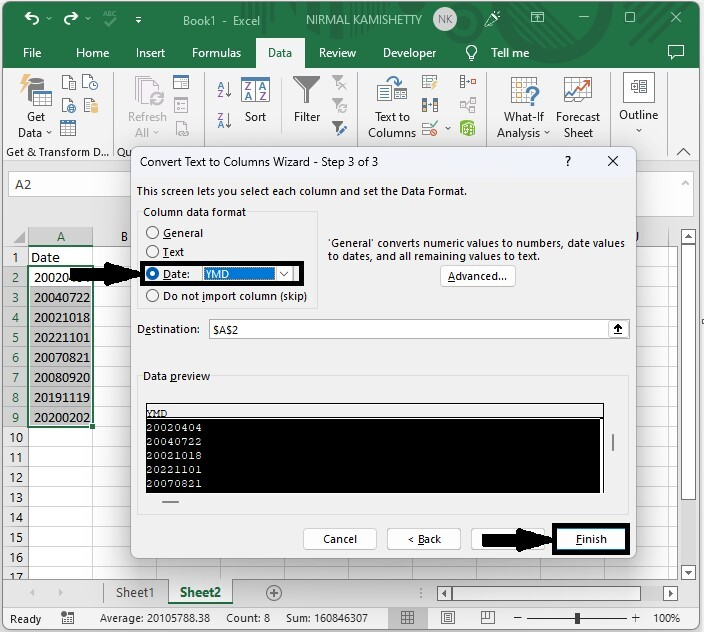## Conclusion

In this tutorial, we used a simple example to demonstrate how you can convert yyyymmdd to normal format in Excel.

Updated on: 07-Mar-2023

9K+ Views